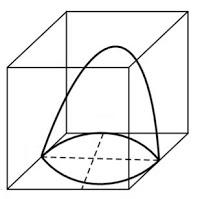Newbie

# A hemispherical depression is cut out from one face of a cubical wooden block such that the diameter l of the hemisphere is equal to the edge of the cube. Determine the surface area of the remaining solid. Q.5

• 0

What is the solution for class 10 ncert chapter surface areas and volumes . Find the best solution of the exercise 13.1 question number 5 give me the easiest and simplest solution of this question .A hemispherical depression is cut out from one face of a cubical wooden block such that the diameter l of the hemisphere is equal to the edge of the cube. Determine the surface area of the remaining solid.

Share

1. The diagram is as follows:Now, the diameter of hemisphere = Edge of the cube = l

So, the radius of hemisphere = l/2

∴ The total surface area of solid = surface area of cube + CSA of hemisphere – Area of base of hemisphere

TSA of remaining solid = 6 (edge)2+2πr2-πr2

= 6l2 πr2

= 6l2+π(l/2)2

= 6l2+πl2/4

= l2/4(24+π) sq. units

• 0